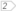#Boost C++ Libraries

...one of the most highly regarded and expertly designed C++ library projects in the world.

##### BOOST_GEOMETRY_REGISTER_POINT_2D

Macro to register a 2D point type.

###### Description

The macro BOOST_GEOMETRY_REGISTER_POINT_2D registers a two-dimensional point type such that it is recognized by Boost.Geometry and that Boost.Geometry functionality can used with the specified type.

###### Synopsis

```#define BOOST_GEOMETRY_REGISTER_POINT_2D(Point, CoordinateType, CoordinateSystem,
Field0, Field1)```

###### Parameters

Name

Description

Point

Point type to be registered

CoordinateType

Type of the coordinates of the point (e.g. double)

CoordinateSystem

Coordinate system (e.g. cs::cartesian)

Field0

Member containing first (usually x) coordinate

Field1

Member containing second (usually y) coordinate

`#include <boost/geometry/geometries/register/point.hpp>`

CautionUse the macro outside any namespace
NoteA point can include a namespace
###### Examples

Show the use of the macro BOOST_GEOMETRY_REGISTER_POINT_2D

```#include <iostream>
#include <boost/geometry.hpp>
#include <boost/geometry/geometries/register/point.hpp>struct legacy_point
{
double x, y;
};

BOOST_GEOMETRY_REGISTER_POINT_2D(legacy_point, double, cs::cartesian, x, y)int main()
{
legacy_point p1, p2;

namespace bg = boost::geometry;bg::assign_values(p1, 1, 1);
bg::assign_values(p2, 2, 2);

double d = bg::distance(p1, p2);

std::cout << "Distance: " << d << std::endl;

return 0;
}
```

 Somewhere, any legacy point struct is defined The magic: adapt it to Boost.Geometry Point Concept Any Boost.Geometry function can be used for legacy point now. Here: assign_values and distance

Output:

```Distance: 1.41421
```